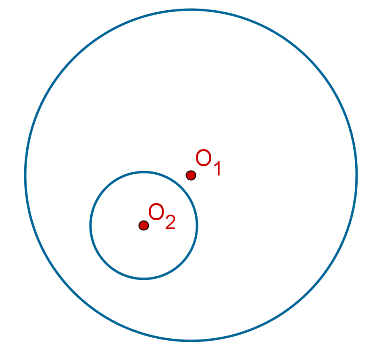# Common Tangents

Go back to  'Circles-Tangents'

Learn from the best math teachers and top your exams

• Live one on one classroom and doubt clearing
• Practice worksheets in and after class for conceptual clarity
• Personalized curriculum to keep up with school

We have already seen that for two circles touching each other (either internally or externally), we can draw a common tangent at the point of contact of the two circles. Now, we will analyze common tangents in more detail.

Suppose that two circles lie externally to each other, touch each other externally, or intersect each other in two distinct points. In these cases, exactly two direct common tangents will exist, as shown in the following diagrams: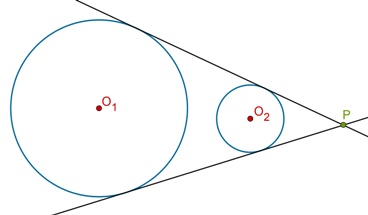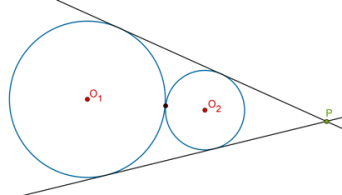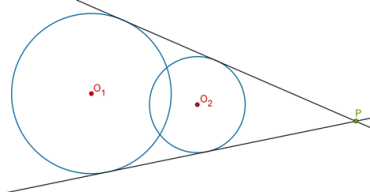The adjective direct means the following: for a direct common tangent, the two circles will lie on the same side of the common tangent.

Now let us consider transverse common tangents. A common tangent is called transverse if the two circles lie on opposite sides of it. In the following situation, we have two circles lying externally to each other, and exactly two transverse common tangents: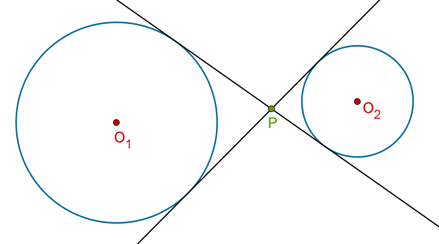For two circles touching each other externally, there will be exactly one transverse common tangent (and of course, two direct common tangents):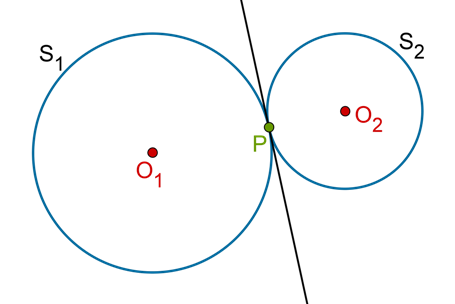For two intersecting circles, there is no transverse common tangent (but two direct common tangents, as we have seen). Similarly, for two circles touching each other internally, there is no transverse common tangent (but exactly one direct common tangent).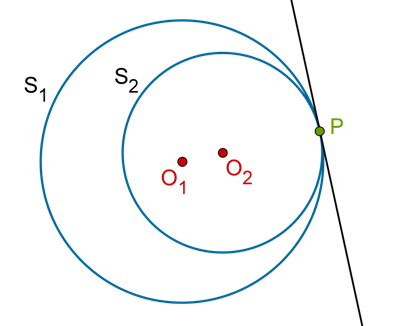Finally, are there any common tangents when one circle lies completely within the other, as in the following figure?